# Mathematic Modeling

## 1. General Description

There were two major goals with regards to our modeling section. To start with, we used image processing to deal with the fluorescence photo and polynomial fitting to detect the moving pattern of MSCs in the Inflammatory Bowel Disease (IBD) mice after they were injected modified MSCs. In the second place, we hoped to predict the living conditions of our IBD mice considering its two obvious advantages, testing whether our modified MSCs were useful and applied this model in the future study, as IBD was not an illness which could be cured thoroughly and we definitely would do deeper research in this career. To be specific, after preprocessed the various experimental data we got, we sifted the interrelate factors and their changing tendency by doing Principal component analysis, we rationalized this process and its results. We analyzed the optimal features and used SVM (Support Vector Machines) and PB (Neural Networks) to do the prediction.

## 2. Modeling Details

· Part 1: Determine the path
As we added Luciferase gene in the MSCs, it was easy to take photos to show the location of modified MSCs in mice in vivo. In these pictures, which would be shown later, higher fluorescence intensity indicated more MSCs concentrated. However, such a method has some restrictions. First, we could only take photos, rather than a video of mice because of the limitation of our equipment. Therefor one could not easily tell a moving pattern of MSCs, only from several of these discreet pictures. Moreover, when taking different photos, those mice were in different positions in the screen. Which made it more difficult to understand the moving pattern. Image processing and polynomial fitting could help to avoid these defects.

i. Moving the mice to the same position.
We took photos of the mice after we injected MSCs into them by 5 min, 0.5h, 1h, 2h, 4h, 8h, 16h, 24h, 32h, 40h and 48h. Those pictures are shown below. Our machine could produce a reference standard besides these pictures.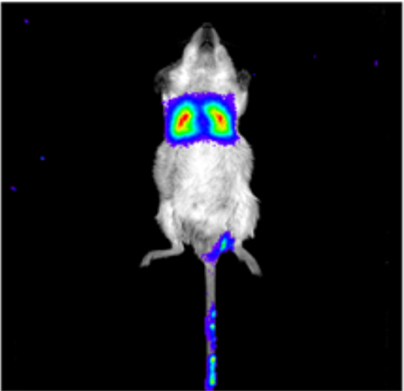Figure 7.1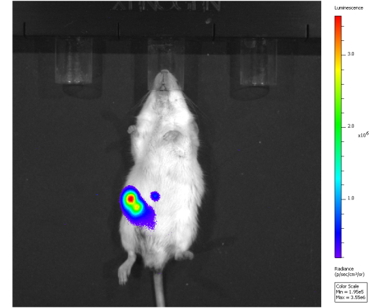Figure 7.2
As you could see, the color in the picture represented the fluorescence intensity and hence, the concentration of MSCs. A brighter color means a high density and the bluer color represented less MSCs concentrated. It was clear that some mice were at the top of the picture and some were at the bottom, making it difficult to gather data.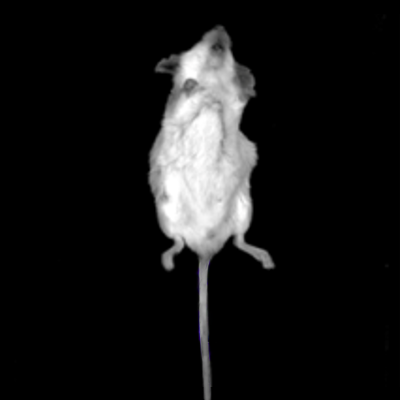Figure 7.3
We chose a somewhat ‘decent’ mouse as the background of showing moving patterns. And then we used MATLAB to process the picture, moving the mouse in different pictures to a relative constant position, which was convenient for further process.

ii. Extracting the fluorescence.
Now we need to extract the fluorescence intensity from the picture. To make this process more clearly, let us consider about the displaying of image first.

At the very beginning, we used gray scale to show image without color. Every pic on the image could be viewed as a function, which equipped every point on the plane with a number, that was, the gray scale. With this notion we could effectively show the basic images without color. But showing color images was a different thing, because color could not be denoted by a single number. Then people introduced the RGB denoting method, which could be viewed as a function equips every point on the plane with a three-dimension vector. Each component of these vectors was a number denoting the intensity of a specific color, say, Red, Green or Blue. It was reasonable because these three colors were the three primary colors, and every other could derive from the composition of them.

Keeping the above discussion in mind, we could not easily segment the picture by different color, based on the restriction of RGB value. By a glance of the numerical form of the pictures in MATLAB, we defined a pic was ‘Red’ as its RGB value R>160, G<160 and B<160. The definitions of other two colors were quite the same. Noticed that when we defined ‘Red’, we must restrict Green and Blue value as well, because if the RGB value of a pic were relatively the same, then this pic was actually grey in human eyes.

By the criteria above we extracted the color portion on the picture. In real process we actually made an open process on the picture, making sure not to omit the peripheral color. Let us put them in the previously picked background. The result was shown below.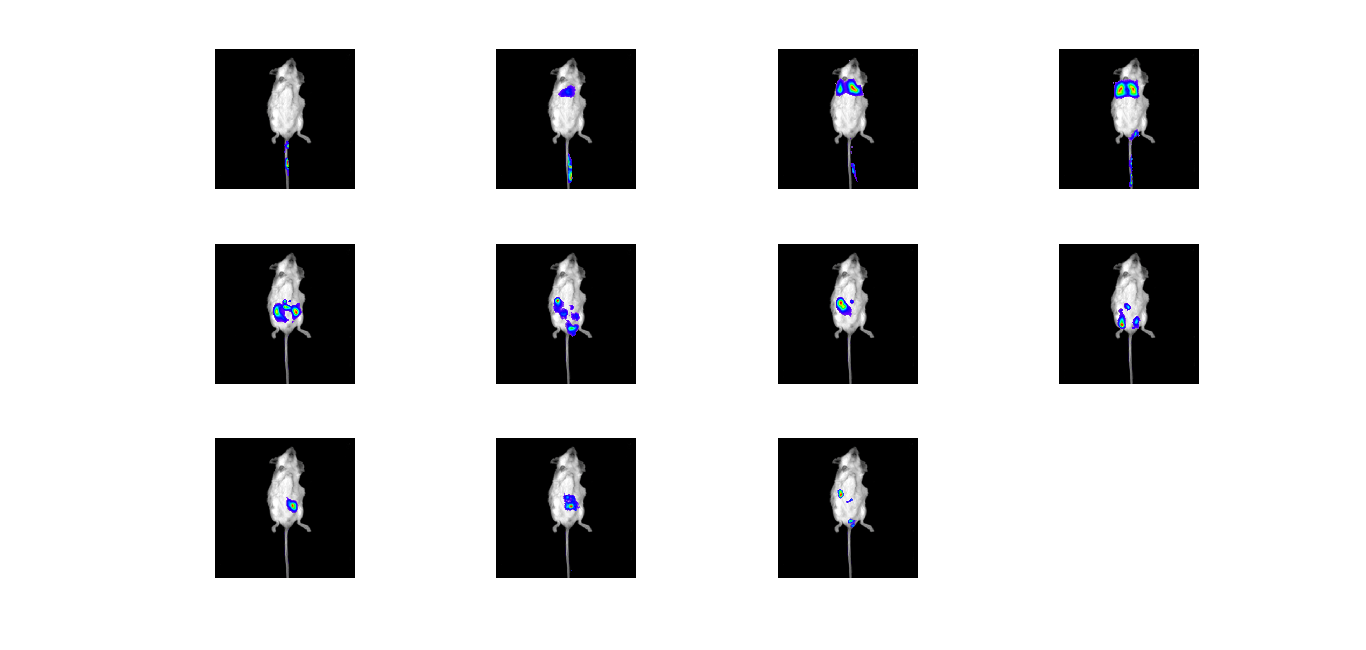Figure 7.4
iii. Fitness.
1) Fit the intensity of fluorescence from color.
By the previous part we knew that the differences of color were due to different RGB values. So we could fit the fluorescence intensity in numerical form from the picture, given the reference standard.
We picked several point on the reference standard with its fluorescence intensity, then we induced the fluorescence of every pic by polynomial fitting.

2) Stimulate the fluorescence changing with respect to time.
Next was the most important part, as we tried to fit the moving pattern of MSCs in mice. We had got the fluorescence from the previous steps, hence we could use the same procedure, for instance, polynomial fitting. For every pic, we got a 13-digit long sequence and each of them denoted the fluorescence on each picture. We used polynomial fitting for every pic about these 13-digit sequences and got a continuously changing pattern of fluorescence.

iv. Making movie.
As the data for a consecutive time had already been generated, the last step for us was making a movie for these pictures. By the way, we added some tab to make the movie clearer and the reference standard to make the movie more understandable. Using the ‘movie2aiv’ function in MATLAB could easily generate a movie. The result was introduced at the modeling part of our project.

· Part 2: Preprocessing & set dataset
i. Standardization.
Standardization of datasets was a common requirement for many machine learning estimators implemented, in principle they could present a bad behavior if the individual features did not more or less looked like standard normally distributed data, for instance: Gaussian with zero mean and unit variance.
Whereas in reality, usually when we did practice, we always ignored the shape of the distribution and just transformed the data to center it by removing the mean value of each features, then scaled it by dividing non-constant features by their standard deviation.
In this case, our raw dataset contained 3 dependent variables: bowel length, weight change rate, DAI scores and features including three major kinds of factors: pro-inflammatory cytokines, anti-inflammatory cytokines, and chemokines collected from mice surviving from 5 minutes to 96 hours after injected modified MSCs.
Considering that our dataset did not own missing values, sparse data or obviously outliers and mean removal, variance scaling would affect the accuracy of medicine examination, thus we merely adjusted the change between natural mice and IBD mice.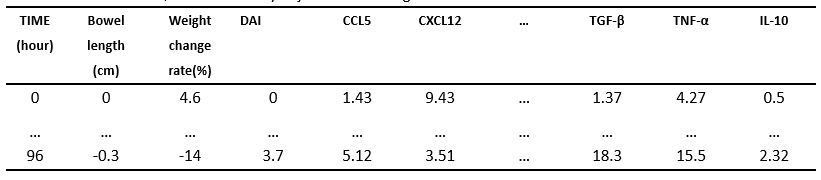ii. Dimensionality Reduction.
As a result of injecting modified MSCs into IBD mice, the bowel length and weight of mice changed dramatically. And we gauged the DAI scores of mice according to their living conditions. The above three variables all can be used to measure the recovering situation of mice.
Nonetheless, with regards to pro-inflammatory cytokines, anti-inflammatory cytokines, and chemokines features as we would have chosen too many to do the judgement, we preferred to find out the most optimal ones to be used in the later machine learning section. If we wanted to get a more thorough analysis, we also had to reduce the number of attributes in dependent variables for summarization.
3) Pearson’s correlation & Spearman’s correlation.
To start with, we checked the relationship among three dependent variables which meant whether they are correlated.
Pearson’s correlation: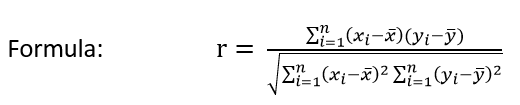Secure Pearson correlation coefficient and p-value in python: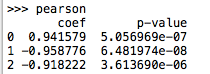Figure 7.5
Plots are shown as below: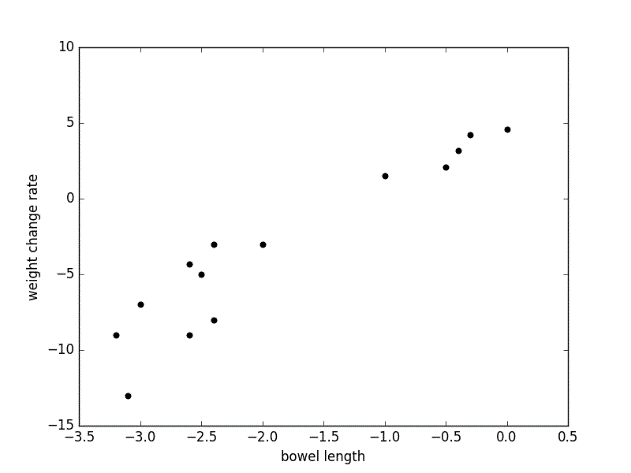Figure 7.6
Fig 1: positive correlation between bowel length and weight change rate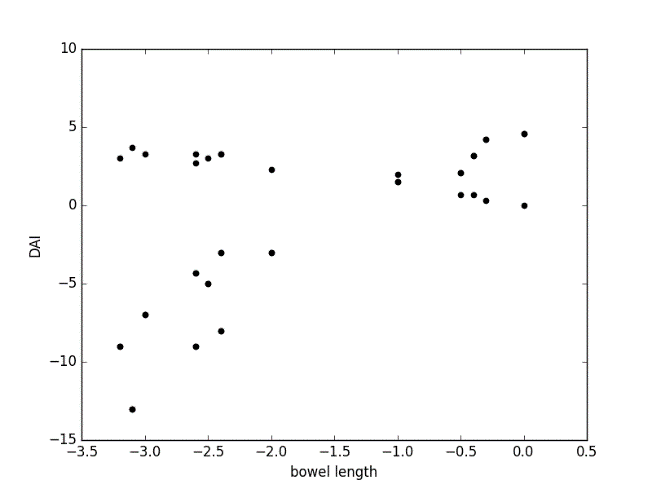Figure 7.7
Fig 2: positive correlation between bowel length and DAI score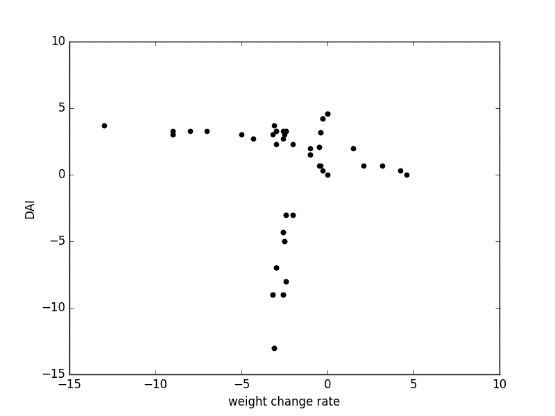Figure 7.8
Fig 3: positive correlation between weight change rate and DAI score

Spearman’s correlation:
As Pearson’s correlation assessed linear relationships, Spearman’s correlation assessed monotonic relationships (whether linear or not). If there were no repeated data values, a perfect Spearman correlation of +1 or -1 occured when each of the variables was a perfect monotone function of the other.
The Spearman correlation coefficient was defined as the Pearson correlation coefficient between the ranked variables.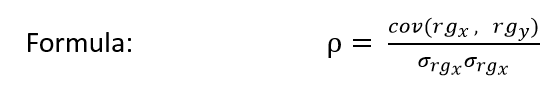cov(rgx, rgy) denotes the covariance of the rank variable
σ(rgx) and σ(rgx) denotes the standard deviations of the rank variables
Secure Pearson correlation coefficient and p-value in python: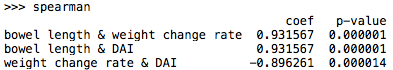Figure 7.9

Plots were analogy to the pictures above.
As the high correlation we achieved, we must come up a method to merge the three variables into a new component.
4) Feature selection using Principal Component Analysis.
PCA was a statistical procedure used an orthogonal transformation to convert a set of observations of correlated variables into another set of linearly uncorrelated variables named principal components the process explained a maximum amount of variance by which way we eliminated the noise and reduce the amount of computation.
Here, we transform the three variables (bowel length, weight change rate, DAI score) into a new component which can then represent the living condition of the IBD mice.

Note: detail information of PCA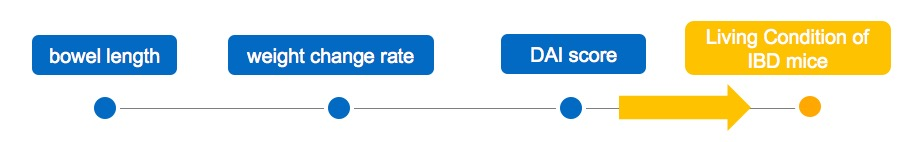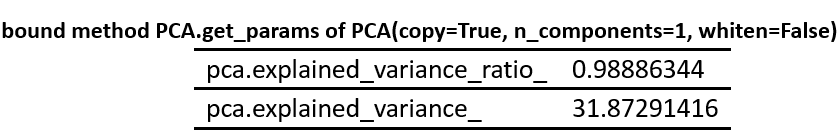The result of PCA:
As the picture showed: the green line was the new component of three other variables.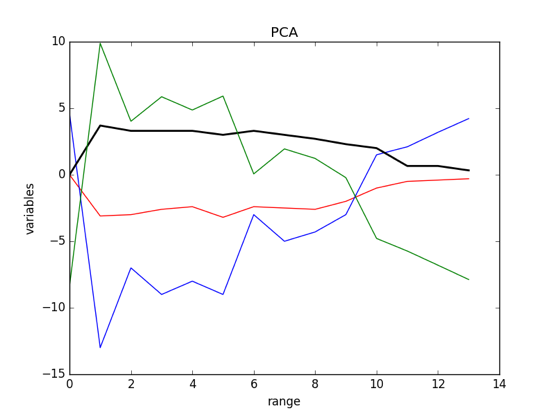Figure 7.10
5) Linear Regression: Ordinary Least Squares.
After got a combined dependent value, we began to consider a way to get optimal features.
There were numerous ways to regularize our model. However, as we did not want to spend time worrying as much about our features being correlated (well, they did highly correlate actually) or with regard to overfitting, we abandoned Na?ve Bayes, Decision Trees and other alternative ways.
Among the 14 features, there were some incredibly relating to the situation of IBD mice, we used regression to pick them out.
OLS is one of the most common ways in Linear Regression, we used this linear model with coefficients
w =(w1, …, wp) to minimize the residual sum of squares between the observed responses in the dataset, and these responses predicted by the linear approximation.
Mathematically it solves a problem of the form: min||XW-y||2^(2)
We used pro-inflammatory cytokines, anti-inflammatory cytokines, and chemokines to approach the final condition.
(1) Unfortunately, we found that the p-value of each parameter was missing, which suggests that our features were highly related and did the regression directly was unwarranted.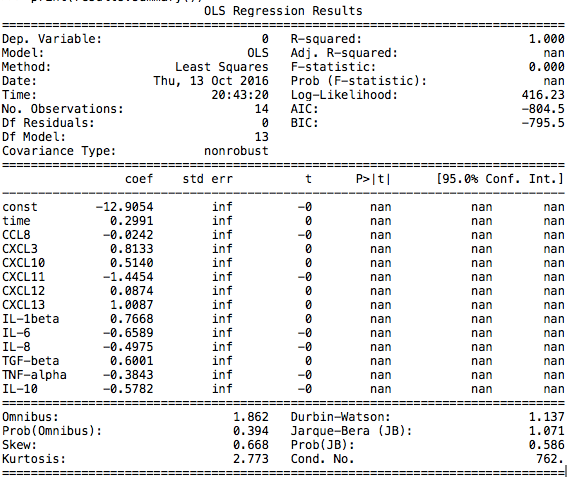Figure 7.11
F(x) = -12.905 + 0.299Xtime – 0.024 XCCL8 + 0.813XCXCL3 + 0.514XCXCL10 – 1.445XCXCL11 + 0.087XCXCL12 + 1.009XCXCL13 + 0.767XIL-1beta – 0.659XIL-6 – 0.498XIL-8 + 0.600XTGF-beta – 0.384XTNF-alpha – 0.578XIL-10
(2) We decided to took advantage of PCA again in that PCA did a fabulous job sorting correlated variables and as PCA used linear combination of all features we did not need to worry about losing details.
We got 8 new components as a result and used OLS again. This time, we were happy to see there was a progress that one of the parameter’s p-value was smaller than 0.05. Thus we eliminated two parameters which own two biggest p-value and did the regression again.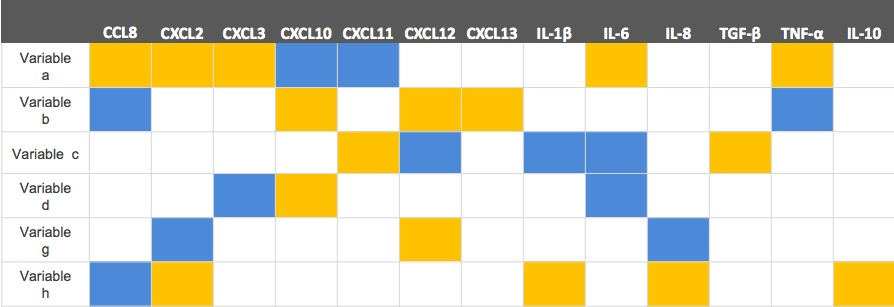Luckily, we found enough parameters whose p-values were smaller than 0.05 and at the mean while their regression coefficients were great.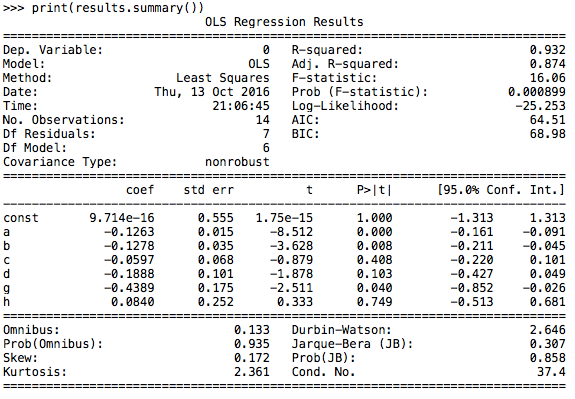Figure 7.12
We ranked the coefficients of various features (whose p-value were smaller than 0.05) and chosen the highest three features in the end.
By doing so, we already got the final reliable dataset.
One thing should be stressed here, when coding the linear regression, the function did not provide the constant of the regression formula, we had to add it by ourselves.
For example:
model = sm.OLS(new_Y, X_test)
results= model.fit()
print(results.params)
print(results.summary())
· PART 3: Machine learning & Supervised Models.

i. Support Vector Machine.
SVMs were a serious of supervised learning methods used for classification, regression and outliers detection.
There was a great deal of advantages of SVMs, for instance, high accuracy even in high dimensional spaces, effective in cases where number of dimensions was greater than the number of samples, nice theoretical guarantees regarding overfitting and with an appropriate kernel they could work well with even if our data was not linear separable in the base feature space, by the way, it was also possible to specify custom kernels. Especially popular in text classification problems where very high-dimensional spaces were the norm.

Note: detail information of SVM
This time, according to our need to predict the situations of IBD mice given their pro-inflammatory cytokines, anti-inflammatory cytokines, and chemokines. We abandoned the popular character of SVM as classifier and acquired its use as Support Vector Regression. There were three different implements of Support Vector Regression: SVR, NuSVR and LinearSVR. LinearSVR provided a faster implementation than SVR but only considers linear kernels, while NuSVR implements a slightly different formulation than SVR and LinearSVR.
We focused on SVR this time.
With the optimal features we obtained above, we took them as Input and define Target as the living standard of IBD mice. Then we split Input and Target as training and test parts, run into memorable SVM models and predicted other living situation using new input.
We could also get the accuracy of SVR test by comparing the predict target with test target.
Our model was multivariable and could not visualize but if the variables were two dimensional the picture should look like: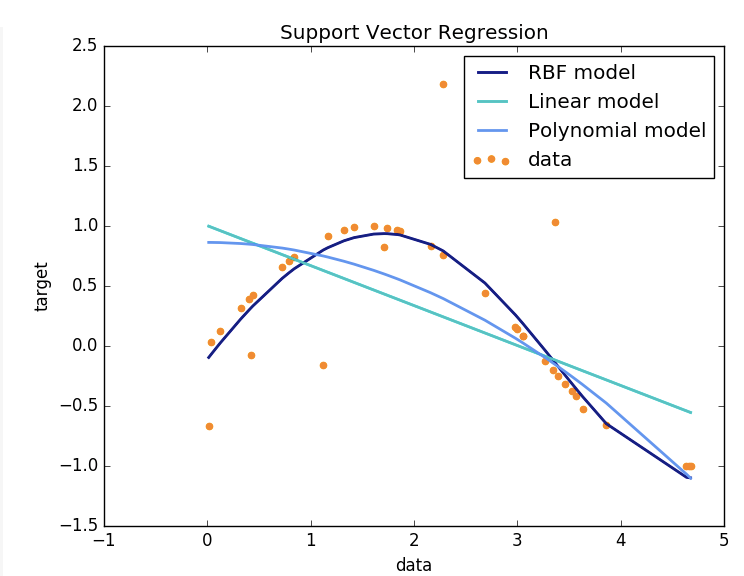Figure 7.13
The codes were like:
X_train, X_test, y_train, y_test = train_test_split(Input, Target, test_size=0.2, random_state=0)
clf = svm.SVR()
clf.fit(X_train, y_train)
clf.predict(X_test)

ii. Neutral Network.
Similar to SVM, Neutral Network including Multi-layer Perception (MLP) which was a supervised learning algorithm that learned a function f(): Rm R0 by training on a dataset, where m was the number of dimension for input and o was the number of dimensions for output. Given a set of features X = x1, x2, …, xm and a target y, it could learn a non-linear function approximator for either classification or regression. While it was different from logistic regression, in that between the input and the output layer, there could be one or more non-linear layers, called hidden layers.
Note: detail information of Neutral Network
Figure 4 showed a one hidden layer MLP with scalar output.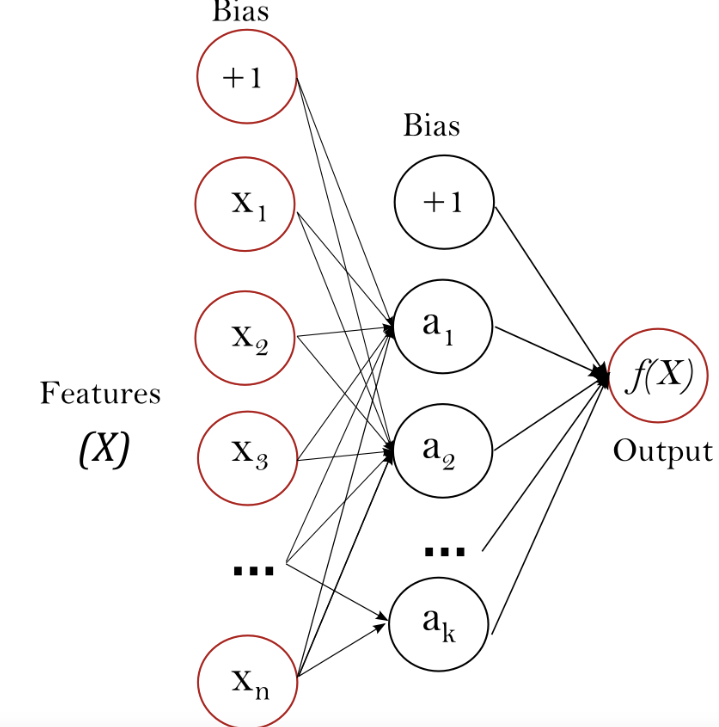Figure 7.14
The rest progresses were similar to progresses using SVM methods.

## 3. Possible improvements

There are actually some defects the prediction of moving pattern of MSCs. First, our fitting polynomial’s order is relatively low. The fitness result is not such reliable, just a raw prediction of the reality. And the color cannot precisely denote the density of MSCs. However, this model is just a simple prediction of the future. In this sense, we this model is very useful.
When processed the data, we realized that our data owned too short time period which meant that the number of observations was quite small. Whereas acknowledged by the public, it was reasonable that the accuracy of machine learning was based on the comprehensiveness of overall data.
In the second place, we used PCA to decrease variables because the high related relationship seriously affected the regression. However, this may not be the optimum method as the process would impair the detailed information the raw data presented.
We would try random forests algorithm in the further analysis and as IBD can not be totally cured even now, we may acquire more chances to develop deeper in this area.

## 4. Reference

1. https://en.wikipedia.org/wiki/Spearman%27s_rank_correlation_coefficient
2. https://en.wikipedia.org/wiki/Principal_component_analysis
3. http://www.statisticssolutions.com/correlation-pearson-kendall-spearman/
4. https://machinelearningmastery.com/deep-learning-with-python/
Deep Learning With Python
Tap The Power of TensorFlow and Theano with Keras,
Develop Your First Model, Achieve State-Of-The-Art Results by Jason Brownlee

## 5. Appendix

Note
i. SVM
In machine learning, support vector machines (SVMs, also support vector networks) are supervised learning models with associated learning algorithms that analyze data used for classification and regression analysis. Given a set of training examples, each marked as belonging to one or the other of two categories, an SVM training algorithm builds a model that assigns new examples to one category or the other, making it a non-probabilistic binary linear classifier. An SVM model is a representation of the examples as points in space, mapped so that the examples of the separate categories are divided by a clear gap that is as wide as possible. New examples are then mapped into that same space and predicted to belong to a category based on which side of the gap they fall on.

In addition to performing linear classification, SVMs can efficiently perform a non-linear classification using what is called the kernel trick, implicitly mapping their inputs into high-dimensional feature spaces.

When data are not labeled, supervised learning is not possible, and an unsupervised learning approach is required, which attempts to find natural clustering of the data to groups, and then map new data to these formed groups. The clustering algorithm which provides an improvement to the support vector machines is called support vector clustering and is often used in industrial applications either when data is not labeled or when only some data is labeled as a preprocessing for a classification pass.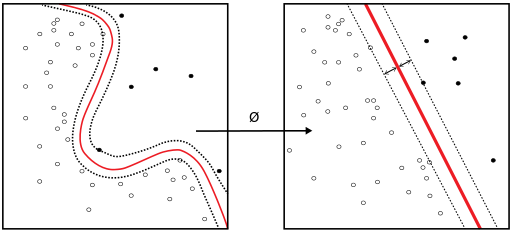ii. Neural Network
Examinations of humans' central nervous systems inspired the concept of artificial neural networks. In an artificial neural network, simple artificial nodes, known as "neurons", "neurodes", "processing elements" or "units", are connected together to form a network which mimics a biological neural network.

There is no single formal definition of what an artificial neural network is. However, a class of statistical models may commonly be called "neural" if it possesses the following characteristics:
1. contains sets of adaptive weights, i.e. numerical parameters that are tuned by a learning algorithm
2. is capable of approximating non-linear functions of their inputs.

The adaptive weights can be thought of as connection strengths between neurons, which are activated during training and prediction.
Artificial neural networks are similar to biological neural networks in the performing by its units of functions collectively and in parallel, rather than by a clear delineation of subtasks to which individual units are assigned. The term "neural network" usually refers to models employed in statistics, cognitive psychology and artificial intelligence. Neural network models which command the central nervous system and the rest of the brain are part of theoretical neuroscience and computational neuroscience.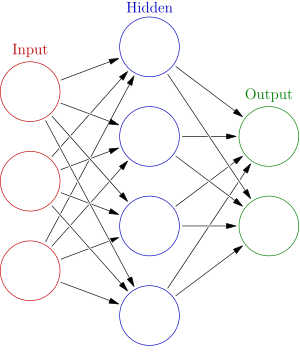iii. Principal component analysis (PCA)
Principal component analysis (PCA) is a statistical procedure that uses an orthogonal transformation to convert a set of observations of possibly correlated variables into a set of values of linearly uncorrelated variables called principal components. The number of principal components is less than or equal to the number of original variables. This transformation is defined in such a way that the first principal component has the largest possible variance (that is, accounts for as much of the variability in the data as possible), and each succeeding component in turn has the highest variance possible under the constraint that it is orthogonal to the preceding components. The resulting vectors are an uncorrelated orthogonal basis set. PCA is sensitive to the relative scaling of the original variables.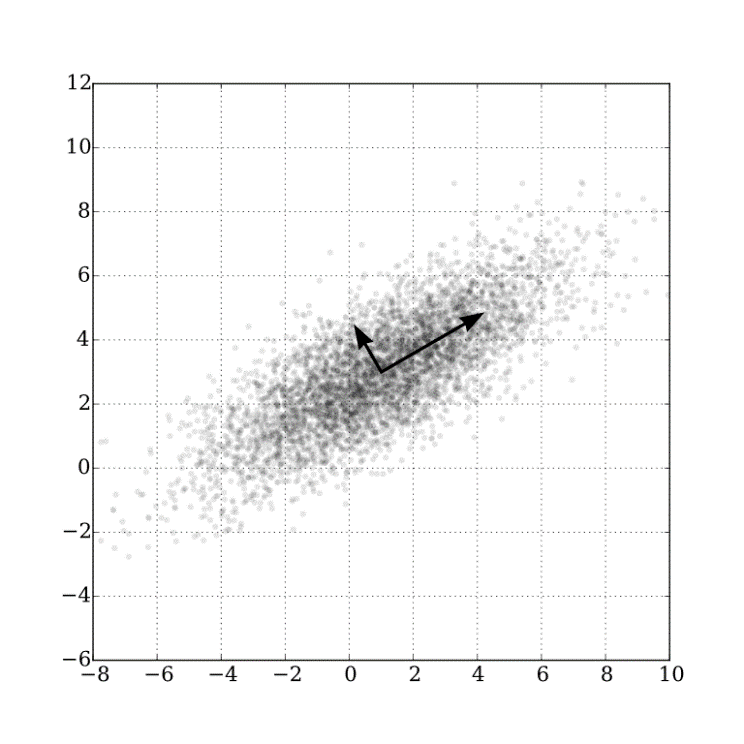Data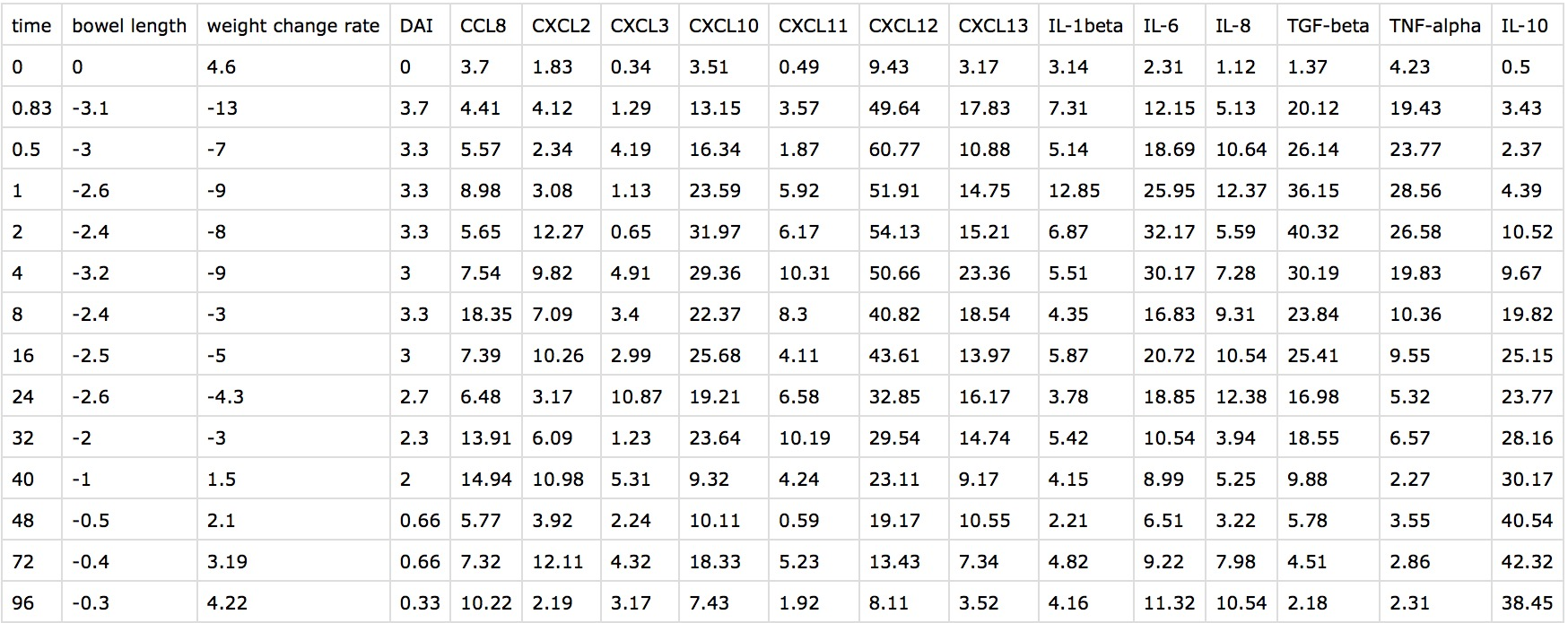Code

```            # -*- coding: utf-8 -*-
"""
Created on Sat Oct 15 10:37:03 2016

@author: yang bai
"""
import pandas as pd
import numpy as np
from sklearn.decomposition import PCA
import scipy.stats as stats
import scipy.optimize as opt
from scipy.stats import linregress
from sklearn import datasets, linear_model
from pandas import DataFrame, Series
import matplotlib.pyplot as plt
import statsmodels.api as sm
from sklearn import svm
from sklearn.svm import SVR
from sklearn.cross_validation import train_test_split
# get data
# correlation test: Pearson & Spearman coef
cor_pearson1 = stats.pearsonr(mice_data['bowel length'],mice_data['weight change rate'])
cor_pearson2 = stats.pearsonr(mice_data['bowel length'],mice_data['DAI'])
cor_pearson3 = stats.pearsonr(mice_data['weight change rate'],mice_data['DAI'])
pearson = []
pearson = list(pearson)
pearson.append(cor_pearson1)
pearson.append(cor_pearson2)
pearson.append(cor_pearson3)
pearson = DataFrame(pearson,columns = ['coef', 'p-value'])
print(pearson)
# plot bowel length & weight change rate
plt.scatter(mice_data['bowel length'], mice_data['weight change rate'], color='black',label = 'Pearson Correlation' )
plt.xlabel('bowel length')
plt.ylabel('weight change rate')
# plot bowel length & DAI
plt.scatter(mice_data['bowel length'], mice_data['DAI'], color='black',label = 'Pearson Correlation' )
plt.xlabel('bowel length')
plt.ylabel('DAI')
# plot weight change rate & DAI
plt.scatter(mice_data['weight change rate'], mice_data['DAI'], color='black',label = 'Pearson Correlation' )
plt.xlabel('weight change rate')
plt.ylabel('DAI')
# Spearman correlation
spearman1 = stats.spearmanr(mice_data['bowel length'],mice_data['weight change rate'])
spearman2 = stats.spearmanr(mice_data['bowel length'],mice_data['DAI'])
spearman3 = stats.spearmanr(mice_data['weight change rate'],mice_data['DAI'])
spearman = []
spearman = list(spearman)
spearman.append(spearman1)
spearman.append(spearman1)
spearman.append(spearman3)
spearman = DataFrame(spearman,columns = ['coef', 'p-value'])
spearman.index = (['bowel length & weight change rate','bowel length & DAI','weight change rate & DAI'])
print(spearman)
# PCA
raw_Y = mice_data.loc[:,['bowel length','weight change rate','DAI']]
pca = PCA(n_components=1)
new_Y = pca.fit_transform(raw_Y)
new_Y = pd.DataFrame(new_Y)
pca.explained_variance_ratio_
pca.explained_variance_
pca.get_params
pca.set_params
# plot
plt.figure()
time1 = range(0,14)
plt.plot(time1,mice_data['bowel length'],'r',label = 'bowel length')
plt.plot(time1,mice_data['weight change rate'],'b',label = 'weight change rate')
plt.plot(time1,mice_data['DAI'],'k',lw =2,label = 'DAI')
plt.plot(time1,new_Y,'g',label = 'component')
plt.title('PCA')
plt.xlabel('range')
plt.ylabel('variables')
# regression
X = mice_data.drop(['bowel length','weight change rate','DAI','CXCL2'], axis = 1)
model = sm.OLS(new_Y, X_test)
results= model.fit()
print(results.params)
print(results.summary())
# PCA
pca1 = PCA(n_components= 8)
new_X = pca1.fit_transform(X)
new_X = pd.DataFrame(new_X)
pca.explained_variance_ratio_
pca.explained_variance_
pca.get_params
pca.set_params
# regression
new_X.columns = ['a','b','c','d','e','f','g','h']
new_X = new_X.drop(['e','f'],axis = 1)
model = sm.OLS(new_Y, X_test1)
results= model.fit()
print(results.params)
print(results.summary())
# SVM
Input = new_X #.drop(['c','d','h'],axis = 1)
Target = new_Y
X_train, X_test, y_train, y_test = train_test_split(Input, Target, test_size=0.2, random_state=0)
# Support Vector Regression
svr_rbf = SVR(kernel='rbf', C=1e3, gamma=0.1)
svr_lin = SVR(kernel='linear', C=1e3)
svr_poly = SVR(kernel='poly', C=1e3, degree=2)
X_train = X_train.as_matrix(columns = None)
y_train = y_train.as_matrix(columns = None)
y_rbf = svr_rbf.fit(X_train, y_train)
predict_rbf = y_rbf.predict(X_test)
y_lin = svr_lin.fit(X_train, y_train).predict(X_test)
y_poly = svr_poly.fit(X_train, y_train).predict(X_test)

```